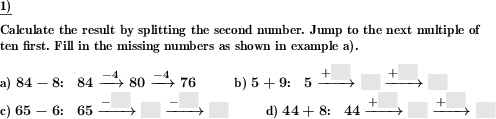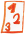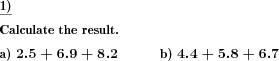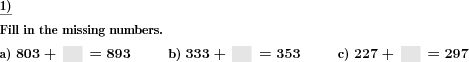Custom math worksheets at your fingertips# Details for problem "Add sub split one digit number"

Quickname: 4896

## Summary

Addition and subtraction by splitting one digit number

## Example## Description

In the number space up to 100, a single digit number has to be added to a two digit number by splitting the single digit number. The first step is to fill up the current tens range, and the second step will go beyond that ten.

A diagram with two arrows representing the two steps illustrates the approach. Two numbers have to be filled in blank spaces for the intermediate result and the end result.

The first problem can be selected to be a sample problem which will be output with the answer.

The number of problems can be selected, and whether addition or subtraction tasks will be shown.

Download free worksheets for this math problem here. The worksheet contains the problems only, the solutions sheet includes the answers. Just click on the respective link.

•Worksheet 1Solution sheet with answers
•Worksheet 2Solution sheet with answers
•Worksheet 3Solution sheet with answers

If you can not see the solution sheets for download, they may be filtered out by an ad blocker that you may have installed. If this is the case, please allow ads for this page and reload the page. The solution sheets will then reappear.

• Do the sample worksheets do not really fit?
• Do you need more math worksheets, with a different level of difficulty?
• Would you like to combine different problems on a worksheet and adjust them to your needs?
• As a teacher, you can put together your own worksheets using the automatically generated math problems provided.
With a free initial credit, you can start creating your own math worksheets in a few minutes.

It does not cost anything to try! Register here, to create custom worksheets now!

## Customization options for this problem

Parameter
Possible values
Number of problems
1, 2, 3, 4, 5, 6, 7, 8, 9, 10
Plus/Minus
Mixed, Plus, Minus
Sample problem
Yes, No

## Other types of problems that appear on worksheets with this problem:

Relevance
Name
Description
Quickname
Example
****
Numbers with or without decimal places have to be added.****
Add multiples of powers of ten
Multiples of powers of ten added to natural numbers.****
Addition powers of ten fill blanks
What multiple of a power of ten has been added?Deutsche Version dieser Aufgabe
These informational pages with samples describe math problems that can be combined on custom math worksheets with solutions for home and school use.
Deutsche Seiten
×